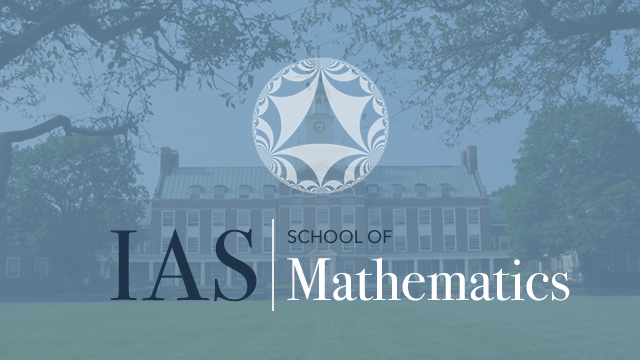## Diophantine approximation with arithmetically small points

February 20, 2018
IAS
Presenters: Vesselin Dimitrov
Length: 1 hour

Watch Video

#### Abstract

Consider a pair $(X,\widehat{L})$ of a regular and projective algebraic variety over $\mathbb{Q}$ and a semipositive adelically metrized line bundle $\widehat{L}$ over $X$. Assume that all of Zhang's successive minima of the pair $(X,\widehat{L})$ are equal, or equivalently, that the infimum of the associated height function $h_{\widehat{L}} : X(\overline{\mathbb{Q}}) \to \mathbb{R}$ is reached by some Zariski-generic sequence. In that setting, I will overview some recent progress on the following question: To what extent does the Galois equidistribution of the small points of $(X,\widehat{L})$ persist when the test function has a logarithmic singularity along some Zariski-closed subset $Z$ of $X$?

At its core this is a Diophantine approximation problem for arithmetically small points and the fixed algebraic target set $Z$. The answer turns out to be affirmative if either the singular set $Z$ is a divisor of minimum height (Chambert-Loir and Thuillier), or if all components of $Z$ are of codimension at least 2 in $X$. We furthermore conjecture that the answer should be negative in every other case. A spinoff of the involved technique leads also to the intersection stability property of the finite unions of minimum height subvarieties of $(X,\widehat{L})$, giving a new approach for the Bogomolov conjecture that applies over both number fields as well as function fields of arbitrary characteristic. Lastly I will mention two consequences of the main Diophantine approximation result, in its most fundamental case where the ambient arithmetic variety is a linear algebraic torus $\mathbb{G}_m^d$ and the approximants are torsion points. These are a quantitative exponential growth rate of the homological torsion in the congruence layers of a fixed abelian $\mathbb{Z}^d$-cover of a manifold, and a quantitative asymptotic of the p-primary part of the homology in the same setting, for arbitrary layers.

Part of this work is joint work with Philipp Habegger.